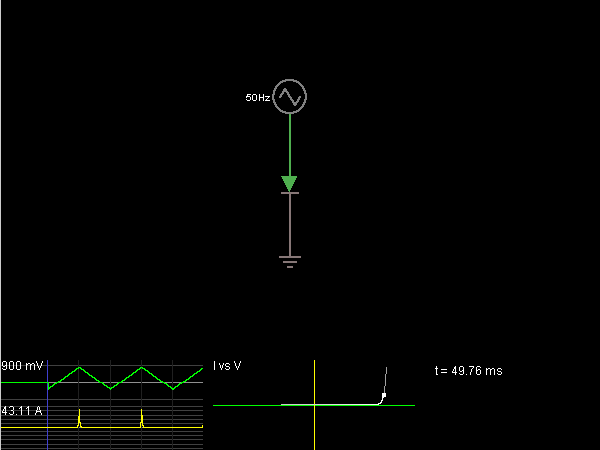# Circuit Simulator - Diode I/V Curve

## Where can I get Diode I/V Curve Circuit Diagram with Explanation?

IndiaBIX provides you lots of fully solved Diode I/V Curve circuit diagram with detailed explanation and working principles.

## How to design a Diode I/V Curve (electronic circuit)?

You can easily design the Diode I/V Curve circuit by practicing the exercises given below. Here you can design and simulate your own electronic circuits with this Online Circuit Designer and Simulator.### Circuit Description:

This example shows the I/V curve of a diode. With a resistor, I (current) and V (voltage) are proportional (by Ohm's Law). With a diode, I and V have an exponential relationship. At the lower left, voltage is shown in green, and current in yellow. At the lower right is a graph of current versus voltage (the I/V curve). -- Credits: Mr. Paul Falstad.